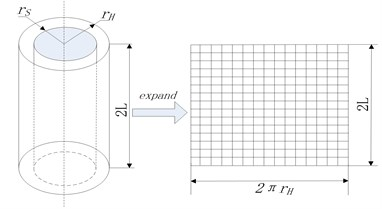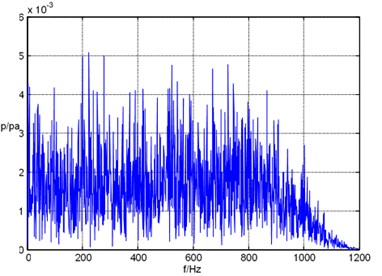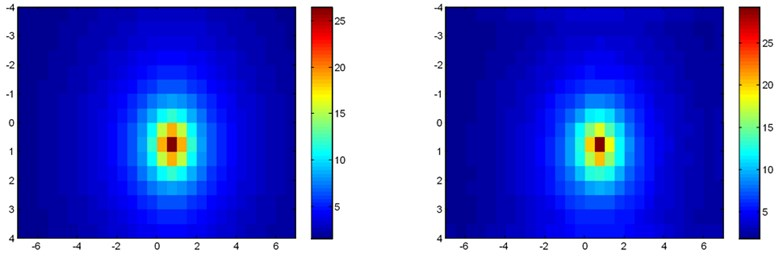Vibroengineering PROCEDIA

Published: 25 April 2019

# Simulation analysis of underwater structure based on local cylindrical acoustic holography

Gaoquan Gu1
Jing Zhang2
Yingchao Shi3
1, 3College of Electronic Engineering, Naval University of Engineering, Wuhan, China
2China Ship Development and Design Center, Wuhan, China
Corresponding Author:
Gaoquan Gu
Views 55

#### Abstract

The local cylindrical acoustic holography technology is introduced, and its application in identifying and locating the column is introduced. The implementation algorithm of local cylindrical holographic reconstruction is given. In engineering operations, columnar sound sources such as electric motors, water pipes, and underwater structures are common noise sources in engineering. Therefore, this paper is of great significance for the location identification and control of such sound sources. The simulation results show that the proposed local cylindrical acoustic holography algorithm is useful to identify the noise source and reconstruct the sound field correctly.

## 1. Instruction

With the development of underwater acoustics and signal processing technology, near-field acoustic holography technology has made great progress. By processing and inversing the information of amplitude, phase and pressure of sound wave, the directivity information of sound field and noise source can be obtained . In theory, near-field acoustic holography algorithms include equivalent source method , boundary element method , and acoustic field space transformation method , among them. The space transformation method of sound field has high computational efficiency and resolution, and its practicability is strong. However, this method requires that the measurement surface and the sound source surface are of the same shape. Domestic scholars have done a lot of theoretical and Experimental Research on this method [5-6], but they all focus on the plane. If the sound source is cylindrical, it will cause greater errors.

For the non-planar sound source, the planar near-field acoustic holography can only obtain the sound field distribution of the equivalent planar sound source on the “virtual source surface” on the reconstructed surface, and can't know the true sound field distribution of the non-planar sound source surface, thus affecting accurate identification and analysis of non-planar sound sources, columnar sound sources or columnar sound sources are sound source structures often encountered in engineering, such as aero engines, electric motors, water pumps and various pipes. For columnar sound source structures, cylindrical near-field acoustic holography must be used to obtain the true sound field distribution of the sound source surface.

According to the above problem, this paper proposes a method of partial cylindrical acoustic holography.

## 2. The realization process of cylindrical acoustic holography

### 2.1. Deduction process

The measurement of the cylindrical near-field acoustic hologram is shown in Fig. 1: the cylinder with the radius of ${r}_{H}$ is the holographic measuring surface, the length of the measuring surface along the axial direction is 2$L$, and the measuring surface is expanded as shown in the right side of the figure; The cylinder with radius ${r}_{S}$ is the reconstruction surface.

Fig. 1Schematic diagram of measurement and reconstruction of cylindrical acoustic holographyAs shown in Fig. 1, the axial measurement interval on the holographic surface is $∆z=2L/\left(2m+1\right)$; The circumferential angle of the measurement is $∆\varphi =2\pi /\left(2N+1\right)$, where $M$ and $N$ are positive integers, the $\left(2M+1\right)×\left(2N+1\right)$ point discrete sound pressure is obtained on the holographic surface. And can be expressed as $-N\le n\le N,-M\le m\le M$. Then, ${p}_{n}\left({r}_{H},{k}_{z}\right)$ discrete space Fourier transform that can approximate the holographic surface sound pressure of $p\left({r}_{H},n∆\varphi ,m∆z\right)$:

1
${p}_{n}\left({r}_{H},i∆{k}_{z}\right)=∆z∆\varphi \sum _{n=-N}^{N}\sum _{m=-M}^{M}p\left({r}_{H},n∆\varphi ,m∆z\right){w}_{2M+1}^{im}{w}_{2N+1}^{jn},$

where $m$ and $j$ are integers from one $M$ to $M$; $n$ and $j$ are integers from one $N$ to $N$; $∆{k}_{z}=\pi /L$; ${w}_{2M+1}$ and ${w}_{2N+1}$ are respectively ${e}^{-2\pi i/\left(2M+1\right)}$ and ${e}^{-2\pi i/\left(2N+1\right)}$. From the discretized ${p}_{n}\left({r}_{H},i∆{k}_{z}\right)$ and Eq. (4):

2
${p}_{n}\left({r}_{S},{k}_{z}\right)\equiv \frac{{H}_{n}^{\left(1\right)}\left({k}_{r}{r}_{s}\right)}{{H}_{n}^{\left(1\right)}\left({k}_{r}{r}_{H}\right)}{p}_{n}\left({r}_{H},{k}_{z}\right),$

the sound pressure cylinder spectrum on the source surface can be approximated as:

3

Therefore, the sound pressure at the discrete points on the reconstructed surface can be obtained by the discrete space Fourier inverse transform:

4
${p}_{n}\left({r}_{s},n∆\varphi \right)=\frac{1}{4\pi L}\sum _{j=-N}^{N}\sum _{i=-M}^{M}pn\left({r}_{s},i∆{k}_{z}\right){w}_{2M+1}^{-im}{w}_{2N+1}^{-jm}.$

From the discretized implementation Eqs. (3), (5) and (6), the cylindrical near-field acoustic holography can be transformed by the computer to obtain the sound pressure field on the reconstructed or predicted surface.

### 2.2. Cylindrical spectral filtering

Wavenumber domain windowing filtering can be used to suppress the amplification process of high spatial frequency component errors. The window function used is similar to the planar near-field acoustic holography, just replace ${k}_{x}$ and ${k}_{y}$ in ${k}_{r}$ with the axial wave number ${k}_{x}$ and the circumferential wave number $n/r$:

5

In the formula, ${k}_{r}=\sqrt{{k}_{z}^{2}+\left(n/r{\right)}^{2}}$; $a$ is the steepness coefficient of the window function; ${k}_{r}$ is the cutoff wave number.

This is a circularly symmetric exponential filter. The choice of ${k}_{c}$ is very important and directly affects the accuracy of the reconstruction results. A method for selecting the holographic surface and the radius of the reconstructed surface and the signal to noise ratio of the holographic measuring system is given below.

Let the sound pressure signal-to-noise ratio of each point of the holographic surface be SNR, ${p}_{nt}\left({r}_{s},{k}_{z}\right)$ is the cylindrical spectrum of the theoretical sound pressure ${p}_{t}\left({r}_{H},{k}_{z}\right)$ of the reconstructed surface with radius ${r}_{s}$, known by Eq. (8):

6
$\frac{{k}_{n}\left({k}_{r}^{1}r\right)}{{k}_{n}\left({k}_{r}^{1}\mathrm{a}\right)}\approx \sqrt{\frac{a}{r}{e}^{-{k}_{r}^{\mathrm{\text{'}}}\left(r-a\right)}}.$

The theoretical sound pressure of the holographic surface with radius ${r}_{H}$ is the relationship between ${p}_{nt}\left({r}_{H},{k}_{z}\right)$ and ${p}_{nt}\left({r}_{s},{k}_{z}\right)$:

7
${p}_{nt}\left({r}_{H},{k}_{z}\right)\approx {p}_{nt}\left({r}_{S},{k}_{z}\right)\sqrt{\frac{{r}_{s}}{{r}_{H}}}{e}^{-{k}_{r}\left({r}_{H}-{r}_{s}\right)}.$

Let ${p}_{nt}\left({r}_{H},{k}_{z}\right)$ be the spiral spectrum of the noise signal component ${p}_{t}\left({r}_{H},\varphi ,\mathrm{z}\right)$ contained in the holographic surface sound pressure, then the cylindrical spectrum $\stackrel{\cup }{p}\left({r}_{H},{k}_{z}\right)$ of the actual sound pressure on the holographic surface can be expressed as the sum of the theoretical sound pressure cylinder spectrum and the noise cylinder spectrum:

8
$\stackrel{\cup }{p}\left({r}_{H},{k}_{z}\right)={p}_{nt}\left({r}_{S},{k}_{z}\right)\sqrt{\frac{{r}_{s}}{{r}_{H}}}{e}^{-{k}_{r}\left({r}_{H}-{r}_{s}\right)+{p}_{ne}\left({r}_{H},{k}_{z}\right)}.$

According to Eqs. (8), the reconstructed surface acoustic pressure spiral spectrum is:

9
$\stackrel{\cup }{p}\left({r}_{S},{k}_{z}\right)={\stackrel{\cup }{p}}_{n}\left({r}_{H},{k}_{z}\right)\sqrt{\frac{{r}_{H}}{{r}_{S}}}{e}^{-{k}_{r}\left({r}_{S}-{r}_{H}\right)}.$

Available from Eqs. (10) and (11):

10
$\stackrel{\cup }{p}\left({r}_{S},{k}_{z}\right)={p}_{nt}\left({r}_{H},{k}_{z}\right)\sqrt{\frac{{r}_{H}}{{r}_{S}}}{p}_{nt}{e}^{-{k}_{r}\left({r}_{S}-{r}_{H}\right)}.$

At this point, the reconstructed surface noise spiral spectrum ${p}_{nt}\left({r}_{S},{k}_{z}\right)$ can be expressed as:

11
${p}_{ne}\left({r}_{S},{k}_{z}\right)=\stackrel{\cup }{p}\left({r}_{S},{k}_{z}\right)-{p}_{nt}\left({r}_{H},{k}_{z}\right)+\sqrt{\frac{{r}_{H}}{{r}_{S}}}{p}_{nt}\left({r}_{H},{k}_{z}\right){e}^{-{k}_{r}\left({r}_{S}-{r}_{H}\right)}.$

To ensure that the reconstruction results are meaningful, it must be ensured that the theoretical sound pressure cylinder spectrum on the reconstructed surface is not overwhelmed by noise, that is, it should be satisfied:

12
$\left|{p}_{ne}\left({r}_{S},{k}_{z}\right)\right|\le \left|{p}_{nt}\left({r}_{S},{k}_{z}\right)\right|.$

According to Eq. (13), it needs to be satisfied:

13
$\left|\sqrt{\frac{{r}_{H}}{{r}_{S}}}{p}_{ne}\left({r}_{H},{k}_{z}\right){\mathrm{e}}^{-{k}_{r}\left({r}_{s}-{r}_{H}\right)}\right|\le \left|{p}_{nt}\left({r}_{S},{k}_{z}\right)\right|.$

According to the previous analysis, in the process of sound wave propagation from reconstruction to holographic surface, the amplitude of the propagation wave is attenuated by the square root of the distance, and the amplitude of the evanescent wave component is attenuated exponentially with the propagation distance. Therefore, the following formula is established:

14
$\sqrt{{k}_{z}^{2}+{k}_{o}^{2}}\le \sqrt{{k}^{2}+\frac{1}{\left({r}_{H}-{r}_{S}{\right)}^{2}}{\left[\frac{SNR1n\left(10\right)}{20}+1n\left[\sqrt{\frac{{r}_{S}}{{r}_{H}}}\right]\right]}^{2}}={k}_{q}.$

Eq. (16) shows that as long as the wavenumber component of $\sqrt{{k}_{z}^{2}+{k}_{o}^{2}}>{k}_{q}$ in the cyber surface acoustic pressure cylinder spectrum is filtered out, it can be ensured that any cylindrical wave component obtained by reconstruction is not overwhelmed by noise. Thus, according to the Eq. (16), it can be determined that the highest wave value used in the reconstruction process is ${k}_{q}$, and this value is used as the cutoff wave number of the wavenumber domain filtering in the reconstruction process.

## 3. Simulation analysis

### 3.1. Simulation conditions and steps

A group of wideband signals is simulated, and the sound field of each frequency point is reconstructed by local aperture acoustic holography algorithm. The sound field reconstruction accuracy of local aperture acoustic holography algorithm is analyzed, and the influence on the calculation of relative local radiated sound power level is evaluated. Sound source: point source, coordinates (0.75, 075); simulation size: ${S}_{1}=$ 28×16 = 13.5 m×7.5 m; array spacing: detal = 0.5 m; the distance of the holographic surface from the sound source: ${Z}_{h}=$ 0.5 m; reconstruction distance from the sound source: ${Z}_{s}=$ 0.4 m; simulation frequency: $f=$ 20 Hz-1 kHz.

### 3.2. Calculation steps

1. Simulate a set of Gaussian noise, through low-pass filtering, the upper limit of the low-pass filter cutoff frequency is 1 kHz.

2. Setting parameters and bringing into the broadband near-field acoustic holography algorithm module.

3.Calculate the reconstructed sound field and relative local radiated sound power level for each frequency point, and the total relative local radiated sound power level of the reconstructed sound field and the theoretical sound field within the bandwidth.

## 4. Conclusion

It can be concluded from the simulation that the local cylindrical acoustic holography can accurately reconstruct the noise source position and the relative local radiated sound power level.

Fig. 2Spectrogram of the first channelFig. 3Theoretical and reconstructed values of the sound field at 0.4 m